# Parallax: reaching the stars with geometry Teach article

How far away are the stars? Explore in your classroom how astronomers measure distances in space.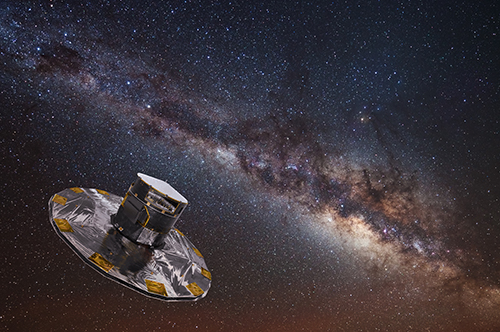Artist’s impression of the Gaia spacecraft, with the Milky Way in the background Image courtesy of ESA / ATG medialab; background image: ESO / S Brunier

Look out of a side window of a moving car or train, and you will notice that your view of objects changes with their distance: nearby bushes or trees seem to be rushing by, while a distant tree or building appears to move far more slowly.

This effect has been used for centuries to determine distances in spacew1. In the mid-19th century, astronomers used parallax to work out the first stellar distances. Surveyors also use this kind of measurement to draw accurate maps of the surface of Earth. Currently, the ESA satellite Gaia, which was launched in December 2013, is measuring extremely accurate parallaxes for more than a billion stars in our galaxy, the Milky Way, increasing accuracy by a factor of about 200.

In this article, we describe an activity that explores the way astronomers use parallax to measure interstellar distances by working out the distance of a ‘star’ set up in a classroom. There is also a short web article about the history of parallax measurements, which you can download from additional material sectionw1.

The activity we describe here reproduces the basic geometry of parallax measurements, using simple devices to measure angles. We have successfully used this activity, which takes between 30 and 45 minutes including setting up, with students aged between 13 and 17. In the next issue of Science in School, we will describe a photographic method of using parallax measurements that is even more accurate and astronomically authentic.

## Activity: the geometry of parallax

For this activity, you will need an instrument for measuring the angles between sight lines – a theodolite (see figure 1), if there is one in your school’s mathematics, physics or geography equipment. If not, we provide instructions here on how to make a similar angle-measuring device from easily available materials.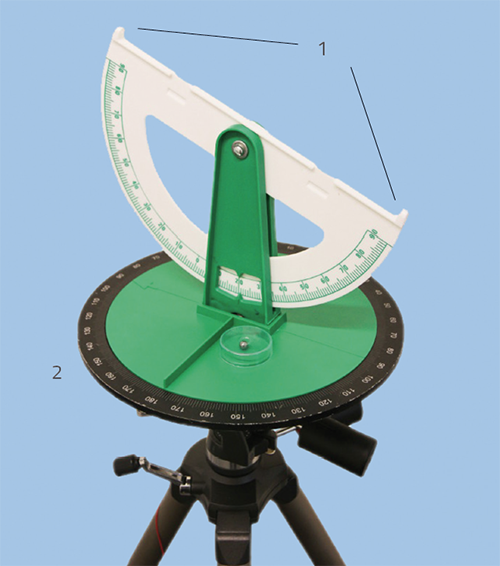Figure 1: A theodolite, with sights (1) and turntable (2) Image courtesy of HdA / M Pössel

### Materials

• Two theodolites
• Small sphere or LED as the ‘star’
• Tape measure

If you do not have access to theodolites, you can improvise by making the simple devices shown in figure 2. For each one (you will need two), you will need:

• Sheet of paper
• Thin, flat cardboard, about 4 cm x 8 cm
• Small wooden block, about 1 cm x 3 cm x 8 cm
• Table or other horizontal surface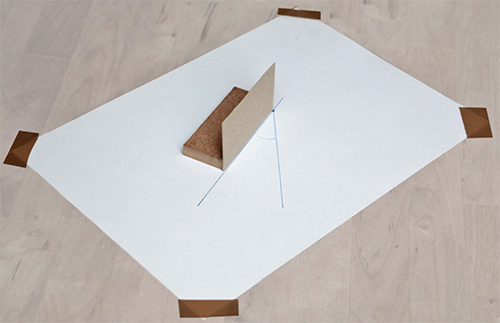Figure 2: Improvised device for measuring angles in a plane, corresponding to the horizontal (azimuth) angle measured by a theodolite Image courtesy of HdA / M Pössel

### Procedure

The basic set-up can be seen in figure 3. To simplify things, we make all the angular measurements in the plane defined by points A, B and C, which should be parallel to the ground.Figure 3: Setup for the angle-measuring activity. The dashed line divides Earth (right) from space (left). Image courtesy of HdA / M Pössel

#### Setting up

1. Divide the room into two sections by marking a line on the ground, as shown by the dashed line. The right-hand section of the room with the two theodolites (or, alternatively, the two improvised devices) represents Earth, while the section on the left represents space.
2. Place the ‘star’ in the space region (position A in figure 3). For the star, you can use an LED, Christmas ornament, ping-pong ball or other small sphere. Mount the star however you can – using a tripod, on a broom handle in an umbrella stand, or from the ceiling.

Follow the set-up steps below if you are using theodolites:

1. Set up your two theodolites, each mounted on its tripod, in the Earth region. You will need to adjust each theodolite’s turntable so that it is completely horizontal (use the spirit level if it has one).
2. Set the declination angle (read from the white semicircular scale in figure 1) to zero. This keeps the sight lines (through the sights in figure 1) within the horizontal plane, in which we will be taking all the angle and length measurements.
3. Adjust the height of the theodolite to be the same as that of the star. You can do this using the telescopic legs of the theodolite’s tripod. With the star some distance from the theodolite, look through the sights and adjust the tripod until the star is directly in a straight-line view. You will probably need to readjust the turntable to make sure it’s still horizontal.

Alternatively, if you are not using theodolites, follow these steps to make the improvised devices:

1. Fix a sheet of paper onto each of the tables or other level surfaces to create two observing platforms corresponding to the theodolite positions B and C in figure 3.
2. Make the ‘sight’ (the line you look along) by firmly fixing the piece of card to the edge of the wooden block, as shown in figure 2.
3. Adjust the height of your star (position A in figure 3) so that it is at exactly the same height above ground as your two sights. Then, from each of your two positions, you should be able to adjust the position of the sights so you can look along the card from the side, exactly edge on, straight towards the star.

#### Taking the measurements

Your task now is to determine the distance between your observing point and the star, taking all your measurements only on Earth. Obviously, you can’t just grab your tape measure and stretch it from B to A, since that would mean leaving Earth. We can’t measure the distances to astronomical objects outside our Solar System by flying there.

Instead, we will measure two angles and the length of one side of the triangle ABC, and geometry will help us to find the length of the remaining two sides, AB and AC. With the theodolite at position B, we can measure the angle ABC as follows:

1. Point the sight of the B-theodolite in the direction of the C-theodolite. Read off the azimuth angle on the azimuth disk (the black scale on the turntable in figure 1). This works best if both theodolites are pointed at each other at the same time.
2. Next, point the sight of the B-theodolite in the direction of the star at A. Again, read off the azimuth angle.
3. Subtract one of the azimuth values from the other. This gives you the value of angle ABC.
4. Repeat the procedure with the theodolite at position C to obtain the angle ACB.
5. Finally, measure the distance between points B and C along the baseline using the tape measure.

With the improvised devices, the same angular measurements can be performed as follows, first for the device at B:

1. Mark a dot on the piece of paper. This will be your reference point.
2. Place the sight so that the lower edge of the cardboard touches the reference point, and the upper edge (along which you are looking) points directly at the other device placed at C. (This works best if the two devices are pointed at each other at the same time, with the upper edges of their sighting cards aligned with each other.)
3. Mark the position of the sight by drawing a line on the paper (as shown in figure 2).
4. Repeat the procedure, looking with the B-device to the star at A, again sighting along the upper edge of the card with the lower edge touching the reference point, and drawing a line along the lower edge.
5. Use a protractor to measure the angle between the two lines you have drawn. This is the angle ABC.
6. Repeat the procedure, using the device at C, looking towards B and A in turn, and marking the sight lines. This will give the angle ACB.
7. Finally, measure the distance between points B and C along the baseline using the tape measure.

#### Finding the star distance

You now know the angles of view of the star from two different positions on Earth, plus the distance between these positions. So how do we use these results to work out the distance to the star? First, look at the geometry of the situation, shown in figure 4.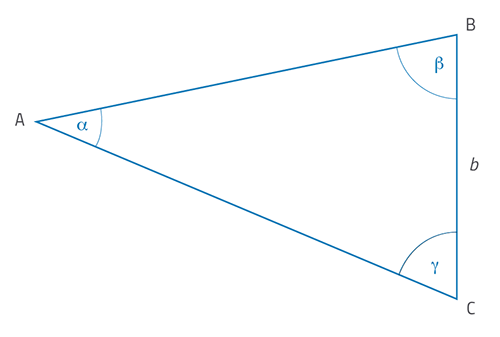Figure 4: Basic geometry of the star and theodolite setup Image courtesy of HdA / M Pössel

In this arrangement, the star position A and the theodolite positions B and C all lie in a horizontal plane and form the triangle ABC (seen directly from above). Angles b and g are the measured angles ABC and ACB, respectively, and the length b is the measured distance between B and C along the baseline.

Using your own measurements, draw a scale diagram of a triangle like this as accurately as possible: a scale of 1:50 on A3 paper gives good results. Then you can simply measure the distances AB and AC from the diagram, and convert these to real distances to determine the distances of B and C to the star A.

To check your results, break the rules! Travel into ‘space’ and use the tape measure to measure AB and BC.

Finally, discuss the accuracy of the results obtained by angle measurement. If we had used larger distances, how would this accuracy change – and why?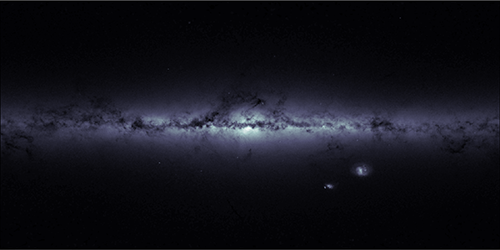The Milky Way galaxy and its neighbours, the Magellanic Clouds, in an image based on data from ESA’s Gaia satellite Image courtesy of ESA / Gaia

## Measurements in space

We hope that students will enjoy a sense of discovery from this activity, and get some idea of the way distance measurements are carried out in astronomyw1. Of course, real astronomical procedures use elaborate methods to ensure the best possible accuracy, because the stars are so very far away and their parallax shifts are so tiny. Even our closest stellar neighbour outside the Solar System (Proxima Centauri) is some 100 000 times further away than the longest baseline distance that can be measured from Earth – that is, twice the distance between the Earth and the Sun, if we take images half a year apart. This is like trying to detect a parallax shift in an object 100 kilometres away when we step one metre to the side.

### Web References

• w1 – Background material on the history of parallax measurements can be downloaded from the additional material section.

### Author(s)

Markus Pössel is Managing Scientist of the Haus der Astronomie (Centre for Astronomy Education and Outreach), and Senior Outreach Scientist at the Max Planck Institute for Astronomy, both in Heidelberg, Germany.

### Review

Stellar parallax is the oldest, simplest and most accurate method for determining the distances to stars. This article explains how to make terrestrial measurements using this method. If you follow the instructions, you will be able to measure the distance within your classroom to an object, the ‘star’, and your students will understand how astronomers can calculate huge distances in space.

Corina Lavinia Toma, Tiberiu Popoviciu Computer Science High School, Romania